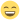## [RESOLVED] Cut-off plots

General help and assistance with jamovi. Bug reports can be made at our issues page: https://github.com/jamovi/jamovi/issues . (If you're unsure feel free to discuss it here)

## [RESOLVED] Cut-off plots

Hello! First of all, thank your for provide us with such an amazing software!

I would like to understand how cut-off plots are made with Jamovi? What is the method used for it?

I am trying to find an optimal cut-off value for an exercise related to a diagnostic test. For this reason, I am using "Binomial Logistic Regression" and the "Cut-off plot" option given by Jamovi inside the last menu called "Prediction".

With this option, the system plots sensitivity and especificity curves, but I do not know why the scale in X axis goes from 0 to 1. I was expecting to have, in X axis, the corresponding scale for the continuous variable in which I am searching for a cut-off value... So that, please, if you can help me to understand how Jamovi does this graphic I will be very grateful!

Kino
Kino

Posts: 3
Joined: Fri Oct 05, 2018 4:23 pm

## Re: Cut-off plots

Hi Kino,

So you are searching for a cut-off value for your dichotomous dependent variable. When you do a binomial logistic regression you can use the model to get a predicted probability for each observation. The predicted probability represents the probability of value 'correct' (or 'yes', or something else depending on the levels in your dependent variable). So this can be a value between 0 and 1.

However, the dependent variable is measured on a dichotomous scale so when you want to predict the values on this scale you need to set a certain cut-off that decides from which probability you're gonna choose "correct". If you set the cut-off value very low, let's say at 0, all outcomes are predicted to be "correct" and sensitivity (i.e., true positive rate) is high while specificity (i.e., true negative rate) is low. When you set the cut-off value very high, let's say at 1, all outcomes are predicted to be "incorrect" and sensitivity is low while specificity is high.

Does that make sense to you?

Cheers,
RaviRavi

Posts: 143
Joined: Sat Jan 28, 2017 11:18 am

## Re: Cut-off plots

Hi Ravi,

Yes, that makes sense for me. But, I would like to understand the following aspects about cut-off plots:

Imagine that you have a continuous variable X, taking values from 0 to 20, and you know that it is a key variable for a diagnostic test. For this reason, you set a first provisional cut-off value, for example, at x=7.5. So, in some sense, you will measure the dependent variable on a dichotomous scale as you have mentioned above; let's say that for values greater than 7.5 you consider that your predicted probabilities are "correct" and for values under or equal to 7.5 are not "correct".

In such a case, if you ask for a cut-off plot to Jamovi -suppose that you have given to the system your continuous variable X and the dependent dicotomic variable-, you obtain a graphic with a cut-off value set at 0.5 by default. My doubts are:

1. Where is located your provisional cut-off value (7.5) for the scale used by Jamovi? How can you give this first cut-off value, set by you, to Jamovi?

2. From the cut-off plot, we can see the cross point between the sensitivity and specificity curves, and from this cross point we obtain a tentative optimal cut-off value. This tentative cut-off value is a number between 0 and 1. I would like to know the optimal cut-off value "translated" to my original scale from 0 to 20.

Am I in a mistake trying to find out this "translation"?

Thank you again!

Cheers,

Kino
Kino

Posts: 3
Joined: Fri Oct 05, 2018 4:23 pm

## Re: Cut-off plots

1. So using the logistic model estimates you can calculate what predicted probability corresponds to any given value of the independent variable. Just fill in the values in the logistic function:Screenshot from 2018-10-06 01-07-58.png (27.62 KiB) Viewed 1498 times
It's even easier to look at the marginal means plot which gives you the predicted probabilities for a range of values of the independent variable.
2. I assume you can calculate this some way, just not sure how. Again, it's easiest to just look at the marginal means plot.Ravi

Posts: 143
Joined: Sat Jan 28, 2017 11:18 am

## Re: Cut-off plots

Hello again Ravi!

Excellent!

Following your last proposal, I have calculated the predicted probability for the tentative optimal cut-off value deduced from the graphic, and it is the value I was expecting for... So, thank you so much for your help!)

My computations did not match with Jamovi's predictions along this afternoon, and now is magic to see how all is ok!

All the best!

Kino
Kino

Posts: 3
Joined: Fri Oct 05, 2018 4:23 pm